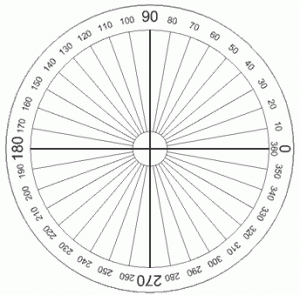# How Many Degrees in a Circle

How many degrees can you get in a circle? It is quite a jokewhen people ask how many degrees can you get in a circle. Of course, if you always get a zero in whatever class you attend you won’t be able to earn any degree. You might have seen this question posted on a comic strip or a paper somewhere, which makes it a bit of a common joke among those who poke around enough.

### How Many Degrees in a Circle

Other than being a joke, what we are taught in school is that there are 360 degrees in a circle. You also have 720 half degrees and so on when we do the math. We also learn early in our lives that 90 degrees make a right angle. All of these things we learn as we go through the growing years and earn our degrees eventually. However, we should take note that mathematicians, engineers, and scientists do not only measure angles in terms of degrees.

### Understanding Degrees

To understand how you get the number of degrees in a circle you should understand what a degree is. A degree is only one of many other units of measurement to represent angles. Other units of angle that you might come across are radians, gradians, square degrees, and steradian. When we deal with a circle expressed in degrees what we are really representing is a full rotation. Each degree therefore represents 1/360 of an entire rotation. Going kind of bit geeky, a degree is the equivalent of Pi divided by 180 radians.

Shifting gears on the discussion of the number of degrees in a circle we then need to understand Pi. Pi, compared to what we usually know about our ever changing world, is a mathematical constant. This basically means this value will never change and the Greek letter Pi is used to symbolize this said value.

To calculate Pi you divide a circle’s circumference by its diameter. It doesn’t matter how large the circle is; given this calculation you’ll end up with the same value for Pi. Calculate that and you get what people usually round off as 3.1416.

However, when do the actual math, you end up with a never ending series of real numbers. Going beyond what we are usually told in school, Pi can be as long as 3.1415926535897932… and so on. It just depends on how determined you are at dividing the numbers over and over.

### Going Beyond Degrees

If you go beyond practical geometry you won’t be satisfied with defining the number of degrees in a circle. Rising above the elementary level of mathematics, you’ll find that angles are usually measured in radians and not in degrees. So, if you’re interested in making the joke a lot more geeky than what it already is, ask how many radians are there in a circle instead of how many degrees in a circle. At any rate, you should expect to get blank stares when people look at you in weird angles so do it at your own risk.